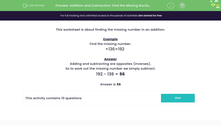# Use Subtraction to Find the Missing Number in a Calculation

In this worksheet, students will use subtraction to find the missing number in an addition.Key stage:  KS 2

Curriculum topic:   Number: Addition and Subtraction

Curriculum subtopic:   Add/Subtract up to Four-Digit Numbers

Difficulty level:#### Worksheet Overview

Example

Find the missing number.

+ 136 = 192

Adding and subtracting are opposites (inverses).

So, to work out the missing number we simply subtract.

192 - 136 = 56

Are you happy to give this a go in the questions that follow?### What is EdPlace?

We're your National Curriculum aligned online education content provider helping each child succeed in English, maths and science from year 1 to GCSE. With an EdPlace account you’ll be able to track and measure progress, helping each child achieve their best. We build confidence and attainment by personalising each child’s learning at a level that suits them.

Get started